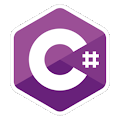Programming Course in C# ¡Free!

# Sum numbers

Proposed exercise

Write a C# program to ask the user for an undetermined amount of numbers (until 0 is entered) and display their sum, as follows:

Number? 5
Total = 5
Number? 10
Total = 15
Number? -2
Total = 13
Number? 0
Finished

Output

Solution

```using System;
public class SumNumbers
{
public static void Main()
{
int number;
int sum = 0;

do
{
Console.Write("Number: ");